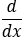# Basic differentiation rules

Definition of derivative, relate it to the slope of the tangent of a curve, derivative of the sum, difference, product and quotient of functions

Differentiation relates to the measurement of change. For a linear function such as y = mx + b where b is the y-intercept and m is the constant slope. Here m is defined as:The tangent line to the graph of y = f(x) at x = c is the line through the point (c, f(c)) with slopeprovided this limit exists.If the instantaneous rate of change of f(x) with respect to x exists at a point c, then it is the slope of the tangent line at that point.The derivative is the slope of the tangent line to a graph f(x) and is usually denoted f’(x).It is calculated by deducing the limit of the difference quotient as ∆x approaches 0. The process of finding the rate at which one variable changes with respect to another is called differentiation.Notation Meaning f’(x) f prime of x y’ y primeA noun, derivative with respect to xA verb, it means to take the derivative with respect to x

## Basic differentiation rules:

Constant ruleHere, c is a constant and the derivative of a constant function is zero.

Ex. f(x) = 5; f’(x)=0

Exponent rulen is a real number. To find the derivative of f (x) = xn.Pull a copy of the exponent out in front of the term and subtract one from the exponent.

Ex. f(x) = x7, f’(x) = 7x6

Constant multiple rulec is a constant and the derivative of a constant times a function is equal to the constant times the derivative of the function.

Ex. f(x) = 3x8 , f’(x) = 3(8x7)=24x7

Sum/difference ruleThe derivative of a sum of functions is the sum of the derivatives and the derivative of a difference of functions is the difference of the derivatives.

Ex. f(x) = 7 + x12 ; f’(x) = 0 +12x11 =12x11

Product ruleEx. f(x) = (x3 + 2x + 5 ) (3x7 -8x2 +1)Quotient Rule

Ex.The chain rule

If h(x) = g(f(x)) then h’(x) = g’(f(x))∙ f’(x). Note that here h(x) is a composite function. In other words, if y = h(x) = g(u), where u = f(x), thenThe chain rule develops the general power rule.

If h(x) = [f(x)]n where n is real then,Ex.Ex.Posted in A2